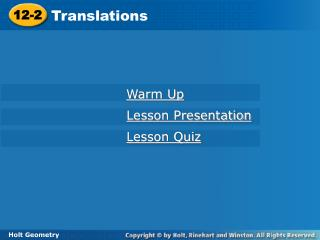Download Presentation12-2

# 12-2 - PowerPoint PPT PresentationDownload Presentation## 12-2

- - - - - - - - - - - - - - - - - - - - - - - - - - - E N D - - - - - - - - - - - - - - - - - - - - - - - - - - -
##### Presentation Transcript

1. Translations 12-2 Warm Up Lesson Presentation Lesson Quiz Holt Geometry

2. Warm Up Find the coordinates of the image of ∆ABC with vertices A(3, 4), B(–1, 4), and C(5, –2), after each reflection. 1. across the x-axis A’(3, –4), B’(–1, –4), C’(5, 2) 2. across the y-axis A’(–3, 4), B’(1, 4), C’(–5, –2) 3. across the line y = x A’(4, 3), B’(4, –1), C’(–2, 5)

3. Objective Identify and draw translations.

4. A translation is a transformation where all the points of a figure are moved the same distance in the same direction. A translation is an isometry, so the image of a translated figure is congruent to the preimage.

5. Example 1: Identifying Translations Tell whether each transformation appears to be a translation. Explain. A. B. No; the figure appears to be flipped. Yes; the figure appears to slide.

6. Check It Out! Example 1 Tell whether each transformation appears to be a translation. a. b. Yes; all of the points have moved the same distance in the same direction. No; not all of the points have moved the same distance.

7. Example 2: Drawing Translations Copy the quadrilateral and the translation vector. Draw the translation along Step 1 Draw a line parallel to the vector through each vertex of the triangle.

8. Example 2 Continued Step 2 Measure the length of the vector. Then, from each vertex mark off the distance in the same direction as the vector, on each of the parallel lines. Step 3 Connect the images of the vertices.

9. Copy the quadrilateral and the translation vector. Draw the translation of the quadrilateral along Check It Out! Example 2 Step 1 Draw a line parallel to the vector through each vertex of the quadrangle.

10.    Check It Out! Example 2 Continued Step 2 Measure the length of the vector. Then, from each vertex mark off this distance in the same direction as the vector, on each of the parallel lines. Step 3 Connect the images of the vertices.

11. Recall that a vector in the coordinate plane can be written as <a, b>, where a is the horizontal change and b is the vertical change from the initial point to the terminal point.

12. D(–3, –1) D’(–3 + 3, –1 – 1) = D’(0, –2) E(5, –3) E’(5 + 3, –3 – 1) = E’(8, –4) Example 3: Drawing Translations in the Coordinate Plane Translate the triangle with vertices D(–3, –1), E(5, –3), and F(–2, –2) along the vector <3, –1>. The image of (x, y) is (x + 3, y – 1). F(–2, –2) F’(–2 + 3, –2 – 1) = F’(1, –3) Graph the preimage and the image.

13. R(2, 5) R’(2 – 3, 5 – 3) = R’(–1, 2) R S R’ S(0, 2) S’(0 – 3, 2 – 3) = S’(–3, –1) U S’ T(1, –1) T’(1 – 3, –1 – 3) = T’(–2, –4) T U’ U(3, 1) U’(3 – 3, 1 – 3) = U’(0, –2) T’ Check It Out! Example 3 Translate the quadrilateral with vertices R(2, 5), S(0, 2), T(1,–1), and U(3, 1) along the vector <–3, –3>. The image of (x, y) is (x – 3, y – 3). Graph the preimage and the image.

14. Example 3: Recreation Application A sailboat has coordinates 100° west and 5° south. The boat sails 50° due west. Then the boat sails 10° due south. What is the boat’s final position? What single translation vector moves it from its first position to its final position?

15. Example 3: Recreation Application The boat’s starting coordinates are (–100, –5). The boat’s second position is (–100 – 50, –5) = (–150, –5). The boat’s final position is (–150, – 5 – 10) = (–150, –15), or 150° west, 15° south. The vector that moves the boat directly to its final position is (–50, 0) + (0, –10) = (–50, –10).

16. Check It Out! Example 4 What if…? Suppose another drummer started at the center of the field and marched along the same vectors as at right. What would this drummer’s final position be? The drummer’s starting coordinates are (0, 0). The vector that moves the drummer directly to her final position is (0, 0) + (16, –24) = (16, –24).

17. 2. Copy the triangle and the translation vector. Draw the translation of the triangle along Lesson Quiz: Part I 1. Tell whether the transformation appears to be a translation. yes

18. Lesson Quiz: Part II Translate the figure with the given vertices along the given vector. 3.G(8, 2), H(–4, 5), I(3,–1); <–2, 0> G’(6, 2), H’(–6, 5), I’(1, –1) 4.S(0, –7), T(–4, 4), U(–5, 2), V(8, 1); <–4, 5> S’(–4, –2), T’(–8, 9), U’(–9, 7), V’(4, 6)

19. Lesson Quiz: Part III 5. A rook on a chessboard has coordinates (3, 4). The rook is moved up two spaces. Then it is moved three spaces to the left. What is the rook’s final position? What single vector moves the rook from its starting position to its final position? (0, 6); <–3, 2>